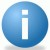# Rounding and Round-off Rules

ByiSixSigma-Editorial

When performing statistical data analyses, quality professionals are always challenged to maintain data integrity. When should you round up the answer; when should you round down? How many significant figures are appropriate for the data set that has been taken?

Below are a set of simple rules that should help you traverse the perils of statistical data analysis.

### Variation Data Round-off

Rule: Round off the answer to one more significant figure than present in the original data.

Rule: This rule is only valid for final results, not intermediate values.

Example: Cycle-time data for an application receipt to account opening process is as follows (in days): 4, 4, 3, 5, 1, 5. The mean of these values is 3.66666666…, and should be rounded to 3.7. Because the original data were whole numbers, we round the answer to the nearest tenth.

### Probability Data Round-off

Rule: Either provide the exact fraction or decimal of the probability or round-off the final result to three significant digits.

Example: The probability of rolling a ‘4’ with a single die is 1/6 or 0.16666666…, which would be rounded-off to 0.167. The probability of a coin landing on ‘tails’ is 1/2 or 0.5 – because 0.5 is an exact figure, it is not necessary to express it as 0.500.

Handpicked Content:   Estimating Sample Size for Process Capability with Special Causes (with Template)

### Sample Size Round-off

Rule: When the calculated sample size is not a whole number, it should be rounded up to the next higher whole number.

Rule: Rounding up a sample size calculation for conservativeness ensures that your sample size will always be representative of the population.

Example: A sample size calculation determined that 2006.083 data points were necessary to represent the population. In this case, 2007 data points samples should be taken.

##### iSixSigma-Editorial

1.2.